Courses

# Points to Remember - Rational Numbers Class 8 Notes | EduRev

## Class 8 Mathematics by Full Circle

Created by: Full Circle

## Class 8 : Points to Remember - Rational Numbers Class 8 Notes | EduRev

The document Points to Remember - Rational Numbers Class 8 Notes | EduRev is a part of the Class 8 Course Class 8 Mathematics by Full Circle.
All you need of Class 8 at this link: Class 8

Points to Remember

A number of the form p/q , where ‘p’ and ‘q’ are any integers and q # 0 is called a rational number.

• Rational numbers are closed under the operations of addition, subtraction and multiplication.
• Rational numbers commutative under addition and multiplication.
• Rational numbers associative under addition and multiplication.
• 4 The rational number ‘0’ is the additive identity for rational numbers, i.e.
[A rational number] + [Its additive inverse] = 0
• The rational number 1 is the multiplicative identity for rational numbers, i.e. [A rational number] x [Its multiplicative inverse OR its reciprocal] = 1
• For all rational numbers, a, b and c, we have:
a(b + c) = ab + ac
a(b – c) = ab – ac
• Rational numbers can be represented on a number line.
• The idea of ‘mean’ helps us to find rational numbers between two given rational numbers.
• We can find an unlimited number of rational numbers between two rational numbers.

WE KNOW THAT
The counting number 1, 2, 3, 4, 5, … are called ‘natural numbers.’ The smallest natural number is 1, but there is no last (or the greatest) natural number. There are infinitely many natural numbers. The number ‘0’ together with the natural numbers gives us the numbers 0, 1, 2, 3, 4, ... which are called ‘whole numbers’. Every natural number is a whole number but every whole number is not a natural number (because ‘0’ is not a natural number). The whole numbers together with the negatives of counting numbers are known as integers. Thus, …, –4, –3, –2, –1, 0, 1, 2, 3, 4, … are integers.
Note:

I. The positive integers are 1, 2, 3, 4, …, i.e. the positive integers are the same as natural numbers.
II. The negative integers are …, –4, –3, –2, –1.
III. The greatest negative integer is –1, and the smallest positive integer is 1.
IV. ‘0’ is neither positive nor negative. It is greater than every negative integer and smaller than every positive integer.

RATIONAL NUMBERS
The signed numbers such as –5, –1, 0, +1, +5, etc. are called ‘integers’. These integers can also be represented as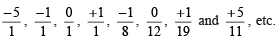We call such numbers as rational numbers. In general, a rational number is a number of the form a/b, where ‘a’ and ‘b’ are any integers, except b may not be zero.Every rational number is the quotient of two integers such that the denominator is a non-zero integer. Thus, the system of rational numbers include all the integers and fractions.

PROPERTIES OF RATIONAL NUMBERS
I . Closure
From our previous knowledge we know that
(a) Whole numbers are closed under

(ii) multiplication
Whole numbers are not closed under
(i) subtraction

(ii) division
(b) Integers are closed under

(ii) subtraction
(iii) multiplication.
Integers are not closed under
(i) division For rational numbers:

For rational numbers:
(i) Rational numbers are closed under addition; i.e. for any two rational numbers ‘a’ and ‘b’, (a + b) is also a rational number.

Example: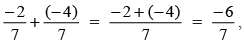which is a rational number.
(ii) Rational numbers are closed under subtraction, i.e. for any two rational numbers ‘a’ and ‘b’, (a – b) is also a rational number.
Example: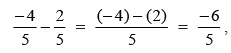which is a rational number.

(iii) Rational numbers are closed under multiplication, i.e. for any two integers ‘a’ and ‘b’, (a × b) is also a rational number.
Example: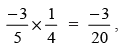which is a rational number.
(iv) Rational numbers are closed under division, if zero is excluded from the collection of rational numbers.
Example: a ÷ 0 is not a rational number.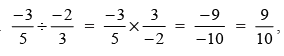which is a rational number.

II. Commutativity
(a) Whole numbers are commutative under

(ii) multiplication
(b) Whole numbers are not commutative under
(i) subtraction

(ii) division
(c) Integers are commutative under

(ii) multiplication
(d) Integers are not commutative under
(i) subtraction

(ii) division
For rational numbers:

(i) Two rational numbers can be added in any order, i.e. the addition is commutative for rational numbers. Thus, for any two rational numbers ‘a’ and ‘b’,
(a + b) = (b + a).
(ii) The subtraction is not commutative for rational numbers.
(iii) Multiplication is commutative for rational numbers. Thus, for two rational numbers ‘a’ and ‘b’,
a x b = b x a.

(iv) Division is not commutative for rational numbers.

III. Associativity
(a) Addition and multiplication are associative for whole numbers.
(b) Subtraction and division are not associative for whole numbers.
(c) Addition and multiplication are associative for integers. (d) Subtraction and division are not associative for integers.
For rational numbers:

(i) Addition is associative for rational numbers, i.e. for any three rational numbers a, b and c,
a + (b + c) = (a + b) + c.
(ii) Subtraction is not associative for rational numbers.
(iii) Multiplication is associative for rational numbers, i.e. for any three rational numbers a, b and c,
a × (b
× c) = (a × b) × c
(iv ) Division is not associative for rational numbers.

THE ROLE OF ZERO (0) AND ONE (1)
(i) The zero is called the identity for the addition of rational numbers, i.e. the sum of 0 and a rational number is the rational number itself.
Note: Zero is also the additive identity for integers and whole numbers as well.

(ii) 1 is the multiplicative identity for rational numbers, i.e. the product of 1 and a rational number is the rational number itself.
Note: 1 is the multiplicative identity for integers and whole numbers also.

NEGATIVE OF A NUMBER
The negative of a rational number a/b, is (-a/b) and the negative of (-a/b), is a/b such that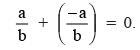Example: The negative of (5/8) is  (-5/8) since, (5/8) + (-5/8) = 0

Note: The negative of a number is also called its ‘additive inverse’.

MULTIPLICATIVE INVERSE
If the product of two rational numbers is 1, then they are called multiplicative inverse of each other.

For example: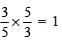Therefore, 5/3 in the multiplicative inverse of  3/5 and 3/5 is the multiplicative inverse of 5/3
Note:
I. The multiplicative inverse of a number is also called the reciprocal of that number.
II. There is no rational number which when multiplied by 0 gives 1.
III. Zero has no reciprocal

DISTRIBUTIVITY OF MULTIPLICATION OVER ADDITION AND SUBTRACTION
For all rational numbers a, b and c,

(i) a(b + c) = ab + ac
(ii) a(b – c) = ab – ac

Solved Questions:
Ques 1: What is the additive inverse of?

(i) -1/13
(ii) 1/5
(iii) 1/-2

Ques 2: What is the multiplicative inverse of?

(i) 51
(ii) -1/3
(iii) 4/-7

Ques 3: What is the product of -6/13 and 7/16 ?

Ques 4: Write four rational numbers between –2 and 0.

Ques 5: Find two rational numbers between 1/2 and 1/5.

Ques 6: Write five rational numbers between 1/3 and 1/5.

Ques 7: Find three rational numbers between (–2) and 5.

Ques 8: Represent -1/2 and 3/4  on a number line.

Ques 9: Represent –1 and -6/5 on a number line.

Ques 10: The product of two rational numbers isIf one of them is -2/3, then find the other.

1. (i) 1/13
(ii) -1/5
(iii) 1/2

2. (i) 1/51
(ii) -3
(iii) -7/4

3. -21/104

4.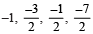5. 7/20 and 11/40

6.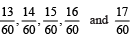7.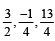10. 14/27

93 docs|16 tests

,

,

,

,

,

,

,

,

,

,

,

,

,

,

,

,

,

,

,

,

,

;